Open in App
Not now

# How Can We Circle Invalid Data in Excel?

• Last Updated : 15 May, 2021

Circle invalid data is used for data validation in Microsoft Excel. To use this go to the Data tab in the menu bar in Microsoft Excel. Before using the circle invalid option you have to write the data validation as per your need.

Let us directly see how we can use the circle invalid data from the starting including the data validation. For the same follow the below steps:

• First of let us see where the circle invalid data option is located.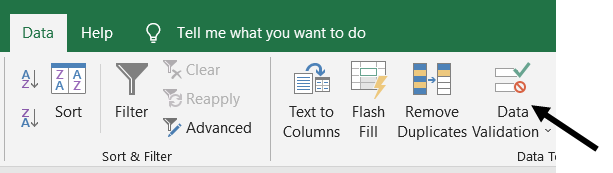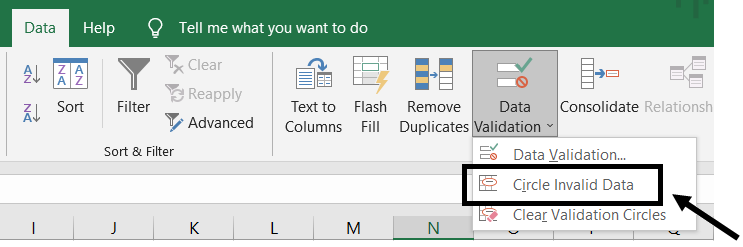• Now let us input some data into the sheet
• We are here considering the marks of the school students and the data validation goes like that if any student has marks less than 33 it is invalid.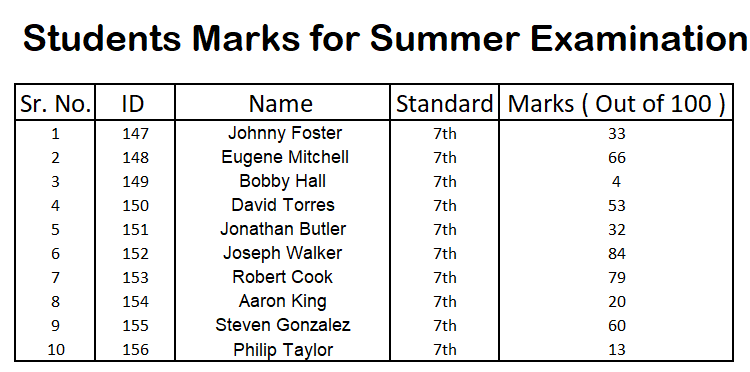• Now for the invalid data, we have to first all need to set the data validation
• So, firstly select the marks column and click on the Data -> Data Validation -> Data Validation.
• Now, you can see the below screen. This is the by default data validation.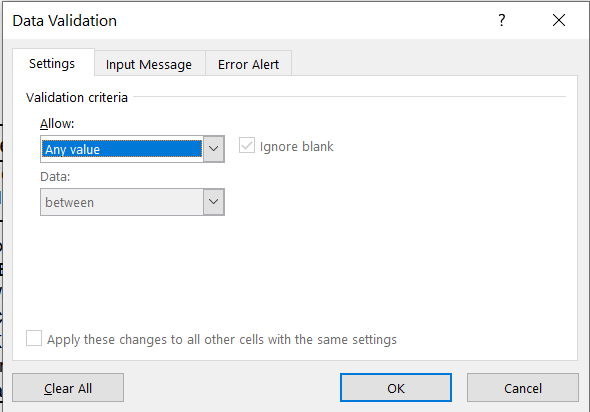• Now you can select only the valid data by selecting the option as per your choice in the Allow section.
• The below image shows all the allowed criteria for the data validation.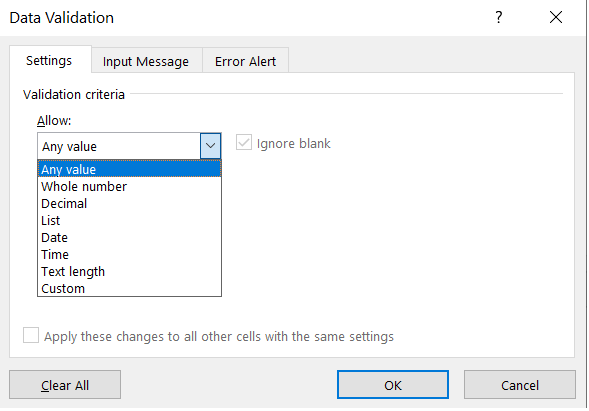• Now for our example, we need the whole number data validation, so we will select that.
• And after selection of the whole number option, another drop-down list will be there as shown below.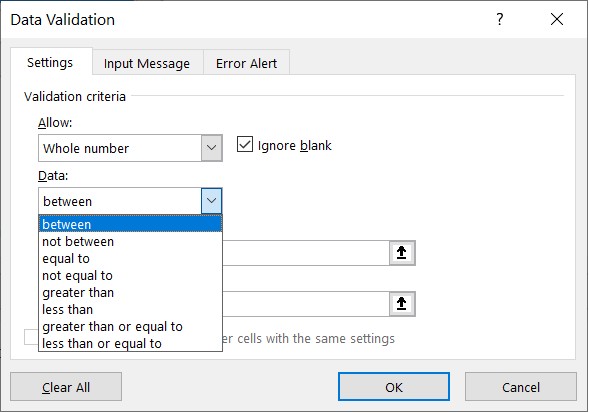• For our example select the greater than option and write down the minimum number that you want ( in our case that is 33 ).
• Here 33 is not included in the valid data. It goes with the invalid data so the validation would be Marks > 33.
• If you want to include 33 then you can go with the greater than or equal to the option.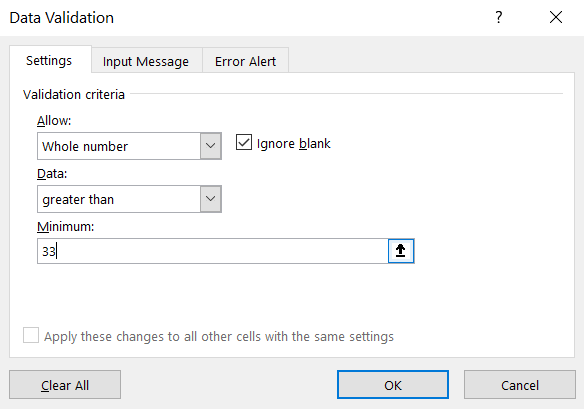• After hitting the ok button select the Data -> Data Validation -> Circle Invalid Data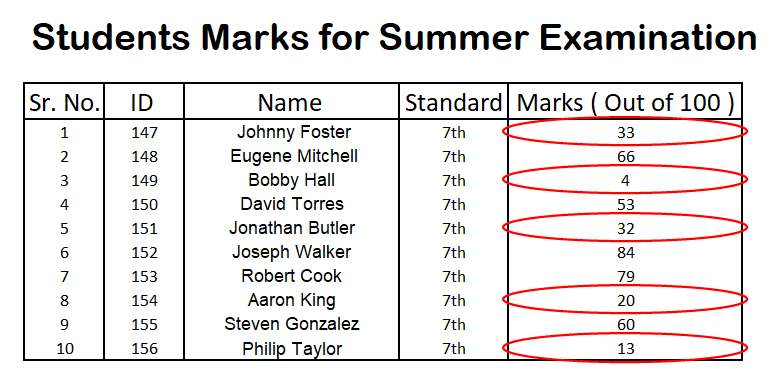• Here all the data which was less than or equal to 33 was marked with the red circle which was invalid as per our example.
• Now if you want to remove this red circle then you must select Data -> Data Validation -> Clear Validation Circles.
My Personal Notes arrow_drop_up